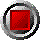Ruthless_Mortgage_Price() functionRuthless_Mortgage_Price() function

Ruthless_Mortgage_Price(argument list…)

This older function values a mortgage security using a Hull-White interest rate tree and asssuming a ruthlessly-exercised (i.e. purely rational and economic, like a callable amortizing bond) prepayment model. MBS_Tabular uses a more sophisticated approach. The function returns the price per \$100 principal.

The function uses the following arguments:

 Argument Description Restrictions WAC the coupon rate on the mortgage security in decimal form, e.g. 8% is expressed as 0.08 (for MBS, this is often lower than the rate on the underlying mortgages) NB: this rate must be specified as an annual rate, with a semi-annual compounding frequency >= 0 Valuation_Date the valuation date for the security (e.g. today); NB: the first element of the zero curve date array must be equal to this value valid Excel date number Maturity_Date maturity date of mortgage valid Excel date number >= Valuation_Date First_Open_Date first "open" date of the mortgage (before this date, full economic prepayment penalties apply, which are based on the entire interest rate differential); if the first open date is past the maturity date, the mortgage is "closed" none Months_PIP the number of months worth of penalty interest for liquidations in the open period (e.g. 3 months is typical for Canada) >= 0.0 RAM the remaining amortization of the mortgage, measured in MONTHS from the valuation date (e.g. 20 years remaining amortization would be entered as 240) > 0 Partials_Percent_per_year is percentage partial prepayments allowed (penalty free) in decimal form, e.g. 15% is expressed as 0.15; NB: assumes that it is percent of REMAINING principal, not original, and also assumes that it is like a liquidation, i.e. no effect on amortization >= 0.0 Swap_Dates array of zero coupon curve dates for the curve (curve should be of comparable credit risk as the mortgages) strictly ascending order The first date of this array must be Valuation_Date Swap_Rates array of continuously compounded zero coupon rates in decimal form (e.g. six percent entered as 0.06) for the curve > 0 correspond to Swap_Rates array Reversion_Rate mean reversion rate of the short rate of interest, in decimal form (Hull-White model) When set to zero, the Hull-White model reduces to the Ho-Lee  model. >= 0 Short_Rate_Vol annual standard deviation of the short rate of interest, in decimal form (Hull-White model) > 0 OAS parallel shift of the zero curve in decimal form none

© 1995-98 Leap of Faith Research Inc.